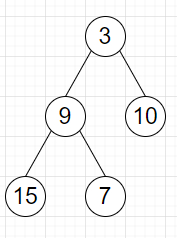# Program to find sum of the right leaves of a binary tree in C++

Suppose we have a binary tree we have to find the sum of all right leaves in a given binary tree.

So, if the input is likethen the output will be 17, as there are two right leaves in the binary tree, with values 7 and 10 respectively.

To solve this, we will follow these steps −

• Define a function dfs(), this will take node, add,

• if node is null, then −

• return

• if left of node is null and right of node is null and add is non-zero, then −

• ret := ret + val of node

• dfs(left of node, false)

• dfs(right of node, true)

• From the main method, do the following −

• ret := 0

• dfs(root, true)

• return ret

Let us see the following implementation to get better understanding −

## Example

Live Demo

#include <bits/stdc++.h>
using namespace std;
class TreeNode{
public:
int val;
TreeNode *left, *right;
TreeNode(int data){
val = data;
left = NULL;
right = NULL;
}
};
class Solution {
public:
int ret = 0;
if(!node)
return ;
ret += node−>val;
}
dfs(node−>left, false);
dfs(node−>right, true);
}
int solve(TreeNode* root) {
ret = 0;
dfs(root, true);
return ret;
}
};
main(){
Solution ob;
TreeNode *root = new TreeNode(3);
root−>left = new TreeNode(9);
root−>right = new TreeNode(10);
root−>left−>left = new TreeNode(15);
root−>left−>right = new TreeNode(7);
cout << (ob.solve(root));
}

## Input

TreeNode *root = new TreeNode(3);
root−>left = new TreeNode(9);
root−>right = new TreeNode(10);
root−>left−>left = new TreeNode(15);
root−>left−>right = new TreeNode(7);

## Output

17